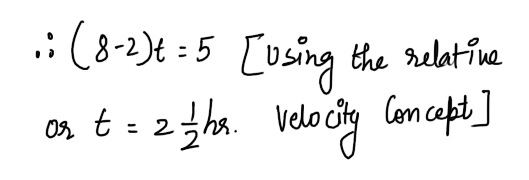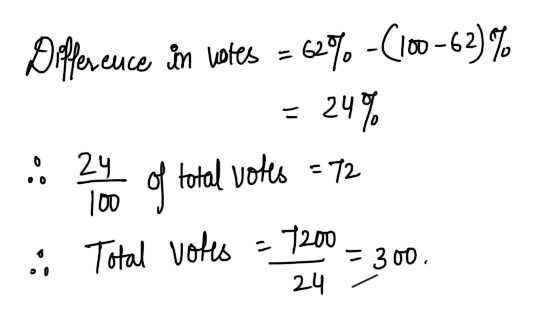# Quantitative Aptitude Quiz for SBI PO Pre - Part 2#### Ques 1.

The numerator and denominator of a fraction are in the ratio of 3 :4 . If 9 is subtracted from the numerator, the resulting fraction has a value 2/3 rd of original Fraction. The numerator of the original fractions is
(a) 27
(b) 18
(c) 36
(d) 9
Ans 1.#### Ques 2.

A man loses 1/2 rd of his money, then wins Rs 10 and then he has exactly what he had at the beginning .What was the amount he originally had ?
(a) Rs 24
(b) Rs 36
(c) Rs 12
(d) Rs 48
Ans 2. Let the man have Rs x initially#### Ques 3.

Satish is 15 km ahead of Ram. The speeds of Satish and Ram are 2 km/hour and 8 km/hour respectively .Ram will overtake Satish in
(a) 5 hours
(b) 3 hours
(c) 11 hours
(d) 2.5 hours
Ans 3. Let the  required time be t hours.#### Ques 4.

To cover a certain distance , a villager cycled half the distance at a speed of 6 km/hour and the remaining half at a speed of 5 km/hour .If the total time taken was 11 hours, the distance covered was
(a) 30 km
(b) 11 km
(c) 60 km
(d) 121 km
Ans 4. Let the required distance be x km.#### Ques 5.

A room is 10 m long, 8 m broad and 6 m high .Its floor is covered with square tiles of sides 20 cm. The number of tiles required to cover the floor is
(a) 480
(b) 400
(c) 2760
(d) 2000
Ans 5.#### Ques 6.

When two numbers 'x' and 'y' are added the sum is 'A'. The larger number is "B' more than twice the smaller. Which of the following is the smaller number ?
(a) (A+2)/2
(b) A + B
(c) ( A - 2)/ 3
(d) ( A- b)/ 3
Ans 6.#### Ques 7.

A man has only 20p coins in his bag. If he has 50 coins inall, totalling Rs 11, how many 20 p coins does he have ?
(a) 25
(b) 30
(c) 20
(d) 22
Ans 7. Let the required number of 25 paise and 20 paise coins be x and y respectively .
. . x + y= 50                                        .....(i)
and x/4 + y/5 = 11 or 5x + 4y= 220    .....(ii)
Solving (i) and (ii), we get  x = 20 , y =30.

#### Ques 8.

How many metres will an insect travel in 18 seconds at a speed of 60 km/hour ?
(a) 108
(b) 1080
(c) 360
(d) 300
Ans 8.#### Ques 9.

Rahul drives his car to his office at a speed of 60 km/hour and returns along the same route at speed of 80 km/hour . His average speed for the entire trip is
(a) 70 km/hour
(b) 68.6 km/hour
(c) 65 km/hour
(d) None of these
Ans 9.#### Ques 10.

& apples and 4 oranges cost as much at 5 apples and 9 oranges .The ratio of the cost of one apple to the cost of one orange is
(a) 5 : 2
(b) 12 : 13
(c) 2 : 5
(d) 3 : 4
Ans 10. Let the cost of an apple be Rs x and  of an  orange be Rs y.#### Ques 11.

If the price of rice is increased by 30%,by how much % should a householder reduce his consumption so as not to increase his expenditure ?
(a) 30%
(b) 16 2/3 %
(c) 23 1/3 %
(d) 25%
Ans 11.#### Ques 12.

A man's speed with the current is 15 km/hour and the speed of the current is 2.5 km/hour . The man's speed against the current is
(a) 12.5 km/hour
(b) 10 km/hour
(c) 8.5 km/hour
(d) 9 km/hour
Ans 12. Man's speed with current = 15 km/hour
Man's speed in still water = 15 -2.5
12.5 km/hour
. . Man's speed against current
= 12.5 - 2.5  = 10 km/hour.

#### Ques 13.

Two trains of equal length are running on parallel lines in the same direction at a speed of 44 km/hr and 38 km/hr respectively .If the faster train crosses the slower train in 48 seconds,length of each train is
(a) 40 m
(b) 82 m
(c) 60 m
(d) 48 m
Ans 13. Let the length  of each train be I metres.#### Ques 14.

At an election. where there are two candidates only, the candidates who gets 62% of the votes is elected by a majority of 72 votes. The total number of votes recorded is
(a) 200
(b) 240
(c) 310
(d) 300
Ans 14.#### Ques 15.

At his usual speed of 48 km/hour , a man reaches his office in time. If he goes by 5/6 th of his usual speed, he will reach his office 8 minutes late. What is distance to his office from home?
(a) 40 km
(b) 32 km
(c) 16 km
(d) 56 km
Ans 15. Let the distance of the man's speed office from his home be x km.